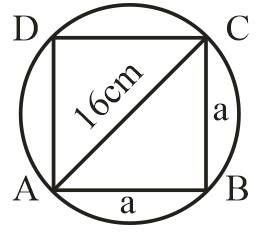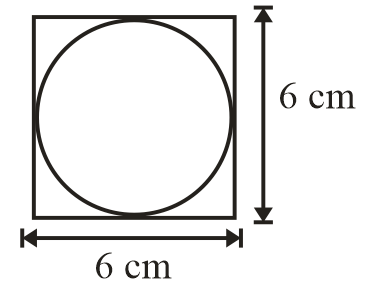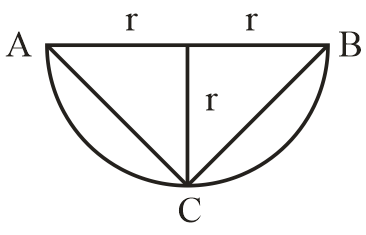Filter By

## All Questions

#### The diameter of a circle whose area is equal to the sum of the areas of the two circles of radii 24 cm and 7 cm is(A) 31 cm                   (B) 25 cm                    (C) 62 cm                    (D) 50 cm

(D) 50 cm

Solution

area of circle =

Radius of first circle (r1) = 24

Area =

Radius of second circle (r2) = 7 cm

Area

Radius of third circle = R

Area of third circle =

According to question

Radius of circle = 25 cm

Diameter =2 R=2 25=50cm

#### The radius of a circle whose circumference is equal to the sum of the circumferences of the two circles of diameters 36cm and 20 cm is(A) 56 cm                   (B) 42 cm                    (C) 28 cm                    (D) 16 cm

(C) 28 cm

Solution

Circumference of circle =

Diameter of first circle (d1) = 36

Diameter of second circle (d2) = 20 cm

Let Radius of 3rd circle = R cm

According to question

## Crack CUET with india's "Best Teachers"

• HD Video Lectures
• Unlimited Mock Tests
• Faculty Support#### The area of the square that can be inscribed in a circle of radius 8 cm is(A) 256 cm2                (B) 128 cm2                (C) 64 cm2             (D) 64 cm2

(B) 128 cm2

Solution

Area of square =a2Diagonal of square = Diameter of circle

Diagonal of square =8 2 =16cm

Let the side of the square = a cm

Using Pythagoras theorem in ABC

(16)2=a2+a2

2a2=256

a2=128

Area of square ABCD= a2

=128 cm2

#### The area of the circle that can be inscribed in a square of side 6 cm is(A) 36 cm2               (B) 18 cm2               (C) 12 cm2               (D) 9 cm2

(D) 9  cm2

SolutionDiameter of circle (d) = 6 cm

Area =            (area of circle = πr2)

## Crack NEET with "AI Coach"

• HD Video Lectures
• Unlimited Mock Tests
• Faculty Support#### It is proposed to build a single circular park equal in area to the sum of areas of two circular parks of diameters 16 m and 12 m in a locality. The radius of the new park would be(A) 10 m                     (B) 15 m                     (C) 20 m                     (D) 24 m

Solution

Diameter of first circle (D) = 16 m

Area =

Diameter of second circle (d) = 12 m

Area =

Let radius of new park = R1

Area =

According to question

R = – 10 is not possible because Radius must be positive.

#### If the perimeter of a circle is equal to that of a square, then the ratio of their areas is(A) 22 : 7                    (B) 14 : 11                     (C) 7 : 22                    (D) 11: 14

Solution

According to question

(Because perimeter of circle = 2πr   Perimeter of square =4 side)

(here side of square =a)

(Using area of square = a2)

Hence ratio of their areas is 14: 11

## Crack JEE Main with "AI Coach"

• HD Video Lectures
• Unlimited Mock Tests
• Faculty Support#### Area of the largest triangle that can be inscribed in a semi-circle of radius r units is(A)   square unit      (B) square unit    (C) 2 square unit     (D) square unit

(A)  square unit

SolutionBase of triangle = diameter of triangle

= 2 x r

Height of triangle = r

#### If the circumference of a circle and the perimeter of a square are equal, then(A) Area of the circle = Area of the square(B) Area of the circle > Area of the square(C) Area of the circle < Area of the square(D) Nothing definite can be said about the relation between the areas of thecircle and square.

(B) Area of the circle > Area of the square

Solution

circumference of a circle=

Let the radius of the circle = r

perimeter of a square =

let the side of a square = a

According to question

circumference of a circle = perimeter of a square

And

Hence Area of the circle > Area of the square.

## Crack CUET with india's "Best Teachers"

• HD Video Lectures
• Unlimited Mock Tests
• Faculty Support#### If the sum of the circumferences of two circles with radii R1 and R2 is equal to the circumference of a circle of radius R, then(A) R1 +R2=R        (B) R1 +R2>R        (C) R1 +R2<R         (D) Nothing definite can be said about the relationamong R1, R2 and R.

(A) R1 + R2=R

Solution

circumference of first circle =2πR1

circumference of second circle =2πR2

Radius of third circle = R

circumference of third circle=2πR

According to question

2πR1 + 2πR2=2πR

2π(R1 + R2)=2πR

R1 + R2=R

Hence option A is correct.

#### If the sum of the areas of two circles with radii R1 and R2 is equal to the area of a circle of radius R, then(A) R1 + R2 =R   (B) R12 + R22=R2  (C) R1 + R2 <R (D)R12 + R22<R2

(B) R12 + R22=R2

Solution

Area of first circle =πR12

Area of second circle =πR22

Radius of third circle = R

Area of third circle=πR2

According to question

πR12 + πR22=πR2

π(R12 + R22)=πR2

R12 + R22=R2

Hence option B is correct.